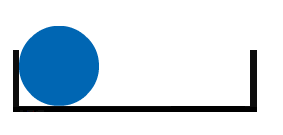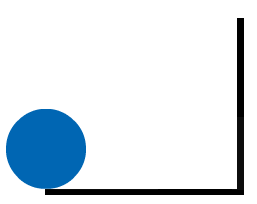#### You may also like### Just Rolling Round

P is a point on the circumference of a circle radius r which rolls, without slipping, inside a circle of radius 2r. What is the locus of P?### Coke Machine

The coke machine in college takes 50 pence pieces. It also takes a certain foreign coin of traditional design...### Just Opposite

A and C are the opposite vertices of a square ABCD, and have coordinates (a,b) and (c,d), respectively. What are the coordinates of the vertices B and D? What is the area of the square?

# Contact

##### Age 14 to 16Challenge Level

Think about a coin with radius $1$ unit in the corner of a box. I roll the coin along the side of the box, making sure it doesn't slip.How long would the box need to be for every point on the coin's circumference to touch the box as I roll it along?

Now imagine the coin rolling along two edges of a box like this:If the two edges are the same length, how long do they need to be to ensure every point on the circumference touches? What if they're not the same length?

Now imagine rolling the coin around on a rectangular tray so that the edge of the coin is always in contact with the inside wall of the tray, and so that it always rolls and never slides. What proportion of the circumference touches the tray as it makes one complete circuit of a $4$ by $3$ tray?

Can you draw any diagrams to help to explain your thinking?

What is the smallest tray you can find where all of the circumference touches the tray at some point?